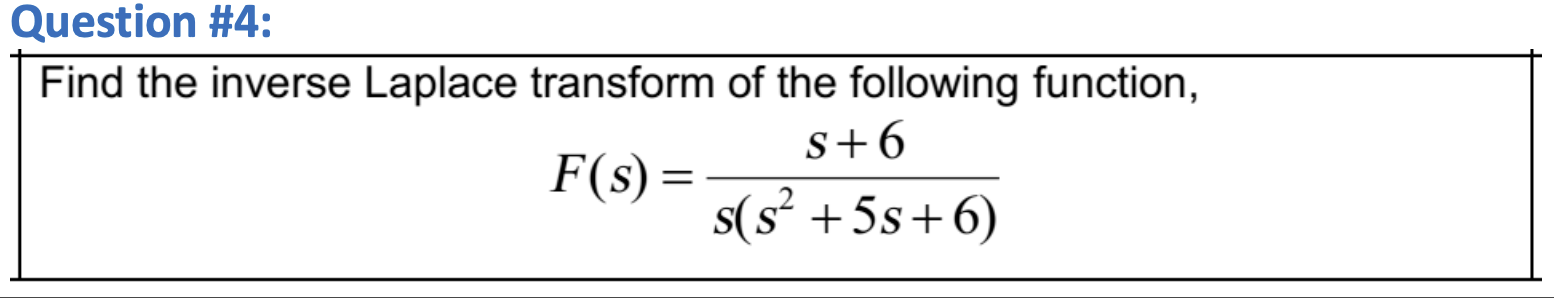Home / Expert Answers / Electrical Engineering / find-the-inverse-laplace-transform-of-the-following-function-f-s-frac-s-pa507

# (Solved): Find the inverse Laplace transform of the following function, $F(s)=\frac{s ...??????? Find the inverse Laplace transform of the following function, \[ F(s)=\frac{s+6}{s\left(s^{2}+5 s+6\right)}$

We have an Answer from Expert